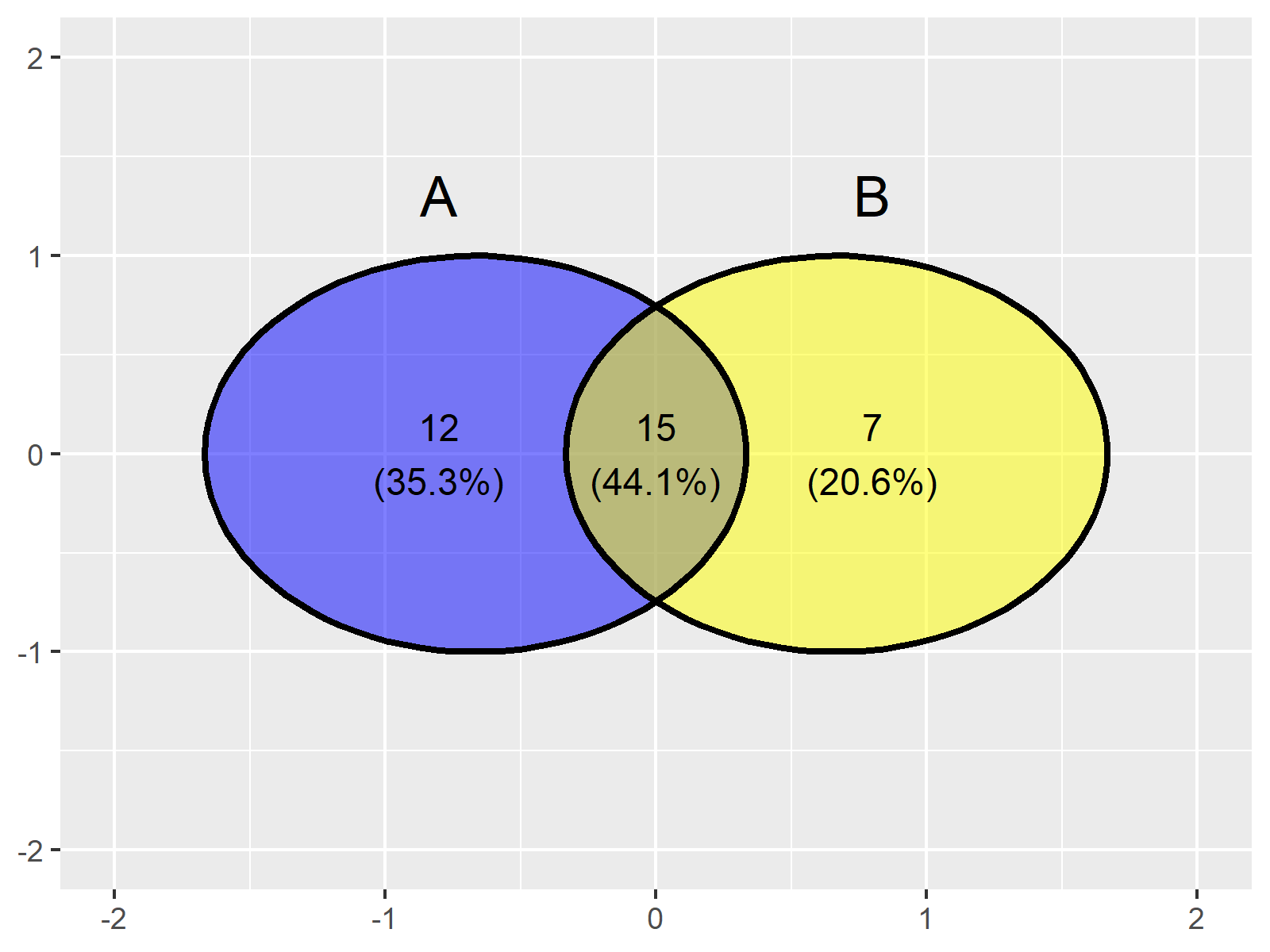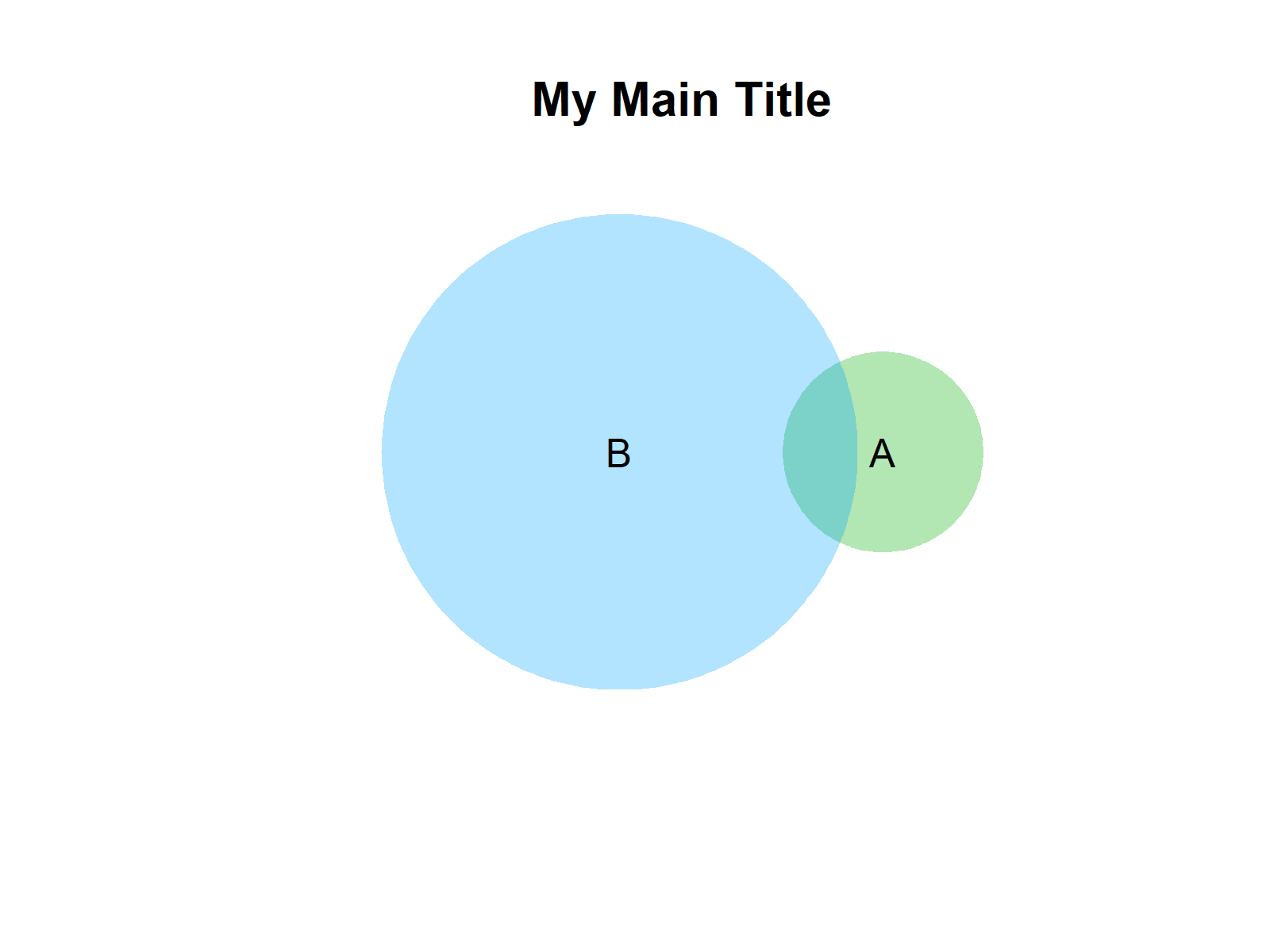# Add Title to Venn Diagram in R (3 Examples)

This tutorial demonstrates how to draw a venn diagram with main title in the R programming language.

Since venn diagrams can be created based on many different packages, I have prepared three examples for three different packages.

The post looks as follows:

Let’s get started.

## Example 1: Add Title to Venn Diagram Created by VennDiagram Package

In Example 1, I’ll illustrate how to draw a venn diagram with titles using the VennDiagram package.

In order to use the functions of the VennDiagram package, we first need to install and load VennDiagram:

```install.packages("VennDiagram")     # Install & load VennDiagram package
library("VennDiagram")```

In the next step, we can use the draw.pairwise.venn function to draw a pairwise venn diagram. Note that we are also creating a plot object called my_venndiag:

```my_venndiag <- draw.pairwise.venn(area1 = 12, # Create venn diagram
area2 = 18,
cross.area = 5)```In the next step, we have to install and load the gridExtra package:

```install.packages("gridExtra")       # Install gridExtra package

We can now use the functions of the gridExtra package to add titles to our plot. In the following R code, I’m using the top argument to add a main title and the bottom argument to add a subtitle:

```grid.arrange(gTree(children = my_venndiag), # Add title & subtitle
top = "My Main Title",
bottom = "My subtitle")```## Example 2: Add Title to Venn Diagram Created by ggvenn Package

The following R syntax demonstrates how to add a main title to venn diagrams created by the ggvenn package.

For this, we first have to create an exemplifying data set that we can use later on to create a ggvenn venn diagram:

```set.seed(68195)                     # Create example data frame
data_venn <- data.frame(value = 1:50,
A = FALSE,
B = FALSE)
data_venn\$A <- data_venn\$value %in% list_venn\$A
data_venn\$B <- data_venn\$value %in% list_venn\$B
#   value     A     B
# 1     1  TRUE FALSE
# 2     2  TRUE FALSE
# 3     3  TRUE FALSE
# 4     4 FALSE  TRUE
# 5     5 FALSE FALSE
# 6     6  TRUE  TRUE```

```install.packages("ggvenn")          # Install ggvenn package

The ggvenn package works in combination with the ggplot2 package, so let’s install and load the ggplot2 package as well:

```install.packages("ggplot2")         # Install ggplot2 package

In the next step, we can use the ggplot and geom_venn functions to draw a pairwise venn diagram:

```ggp <- ggplot(data_venn, # Apply geom_venn function
aes(A = A, B = B)) +
geom_venn()
ggp```Figure 3 visualizes the output of the previous code, a venn diagram without title.

We can now use the typical ggplot2 syntax to add a title on top of our plot:

```ggp + # Add title to ggplot2 venn diagram
ggtitle("My Main Title")```## Example 3: Add Title to Venn Diagram Created by venneuler Package

Another package that can be used to create venn and Euler diagrams is the venneuler package.

We first have to install and load the venneuler add-on package, to be able to use the functions that are contained in the package:

```install.packages("venneuler")       # Install venneuler package

Next, we can use the plot and venneuler functions to create a venn diagram without a main title:

```plot(venneuler(c(A = 10,    # Draw pairwise venn diagram
B = 75,
"A&B" = 4)))```If we now want to append a title above our graph, we can use the main argument of the plot function:

```plot(venneuler(c(A = 10,    # Add title to venn diagram
B = 75,
"A&B" = 4)),
main = "My Main Title")```## Video, Further Resources & Summary

I have recently published a video on my YouTube channel, which explains the contents of this tutorial. Please find the video below.

Please accept YouTube cookies to play this video. By accepting you will be accessing content from YouTube, a service provided by an external third party.If you accept this notice, your choice will be saved and the page will refresh.

Furthermore, you might read the other tutorials on this website. I have published several tutorials already.

To summarize: You have learned in this article how to create a venn diagram with title on top of the plot in the R programming language. If you have additional questions, please let me know in the comments below.

Subscribe to the Statistics Globe Newsletter

•Moritz
March 15, 2023 8:12 am

Hi Joachim,
thanks for the helpful tutorial. I just created a venn diagram with the ggvenn package, but it bothers me that the label of the circles is not centerd over them. Is there a way to change that?
Moritz

•March 15, 2023 9:00 am

Hello Moritz,

Can you share the code please?

Regards,
Cansu

•Moritz
March 15, 2023 9:15 am

Hello Cansu,

sure, sorry about that. Thats my Code:

nh <- list(`with` = c(1:67),
`without` = c(36:79))

ggvenn(nh, c("with", "without"),
digits = 0,
fill_color = c("blue2", "yellow2"),
fill_alpha = 0.6,
set_name_size = 21,
text_size = 18,
stroke_size = 1.5)

I would like to move the two labels 'with' and 'without' over the center of the corresponding circles. I guess it doesn't matter whether the plot is viewed in R or saved to a file? I already tried theme(text = element_text(hjust = .1)), but that didn't do anything.

Thanks for the help,
Moritz

•March 15, 2023 10:08 am

Hello Moritz,

I think they are centered by default. In your case, the text size is the problem if I got your question well. Please see the code below for the Venn diagram with reduced-size text.

```ggvenn(nh, c("with", "without"),
digits = 0,
fill_color = c("blue2", "yellow2"),
fill_alpha = 0.6,
set_name_size = 4,
text_size = 4,
stroke_size = 1.5)```

Regards,
Cansu

•Moritz
March 15, 2023 11:08 am

Hello Cansu,

thanks for looking into it. I made the text that big to have a pixel-free plot after saving it via png(height = 2000, width = 2000). Also I checked if the text appears centerd with the smaller text and for me it still looks a bit off, both after saving and in the plot window. But it is not that important. I was just wondering if it is possible to move it, but it seems to be quite difficult, so I guess I have to live with it.

Thanks again,
Regards,
Moritz

•March 15, 2023 11:35 am

Hello Moritz,

I couldn’t find anything relevant on the internet, unfortunately. Maybe you post your question on our Facebook discussion group, and someone else can help.

Regards,
Cansu

Top